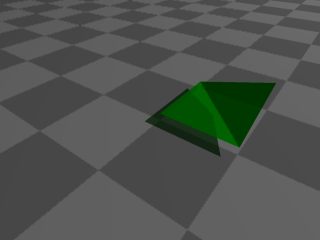# Parametric Surfaces in POV-Ray (work in progress)

By Xah Lee. Date:

This page shows you POV-Ray's syntax for parametric surfaces (math). If you don't know POV-Ray, please see Intro to POV-Ray.

## The “mesh” Patch

The “mesh” primitive is just a list of triangles, as simple as that. Here's a example:3 triangles, made by the “mesh” keyword. Note that triangles need not share vertex or edge. Source: [mesh.pov].

Here's the syntax for the mesh primitive:

```#declare c1 = texture {pigment {color Red}}
#declare c2 = texture {pigment {color Green}}
#declare c3 = texture {pigment {color Blue}}

mesh{
triangle{<0,0,0>,<1,0,0>,<0,0,1> texture { c1 }}
triangle{<1,0,0>,<0,0,1>,<1,.3,1> texture { c2 }}
triangle{<-0.1,0,0>,<-0.1,0,1.2>,<-0.1,1.2,0.7> texture { c3 }}
translate <0,.1,0>
}```

Note that in the mesh primitive, texture must be predefined. This is just how POV-Ray is.

## The “mesh2” Primitive

In a parametric surface, the list of triangles making up the surface share edges and vertexes. If we were to save the surface data as a list of triangles, there will a lot of repetitions of the vertex coordinates. It would be much more efficient, to simply store all the vertexes in a surface as a list, and store another list that specifies which of vertexes are connected to form a triangle.

In POV-Ray, there's a “mesh2” primitive, that stores a surface as a list of vertexes and a list of faces, using indexes of the vertex list. Here's a example:

```mesh2 {
vertex_vectors {
4 /* number of vertexes*/,
<0,0,0>, <1,0,0>, <1,0.3,1>, <0,0,1> // a listing of the vertex
}
face_indices {
2 /* number of faces*/,
<0,1,3>, /*indexes from the vertex_vectors list*/
<1,2,3>
}
}```

In the above, the mesh2 primitive has vertex_vectors{} and face_indices{} as components. In vertex_vectors, it start with the number of total vertexes, following by the vertexes. In face_indices, it start by the number of faces, followed by a set of triangles, each triangle is of the form <v1,v2,v3>, where the v1, v2, v3 are the indexes from the vertex_vectors list, each representing a corner of the triangle.2 triangles, made by the “mesh2” keyword. Note that the triangles are always edge-to-edge, forming a patch of a surface. [mesh2.pov]

work in progress

## IsoSurfaces

POV-Ray has a feature that allows you to plot isosurface. More specifically, you can use it to plot the roots of polynomial of 3 variables.

For example, the equation for a torus is `(R-Sqrt[x^2+y^2])^2 +z^2 - r^2 == 0` where R is the major radius and r is the minor radius.

work in progress